" 行胜于言 "

#  ML-Neural Network# Foreward

It has been a long time after I wrote this note draft due to busy work and other notes on data science.

I am excited by figuring out back propagation algorithm which might be a little bit intricate but intriguing conception in machine learning. To understand it, it would be better to put forward some examples or use visualization tools.

Anyway, this note, which focuses on back propagation algorithm, will be totally finished. And next episode is ongoing.

# Definition/representation

dendrite 树突

nucleus 核

cell body 细胞体

node of Ranvier 郎飞结

axon 轴突

myelin sheath 髓鞘

Schwann cell 许旺细胞

axon terminal 轴突末端

# Neuron model

## Model diagram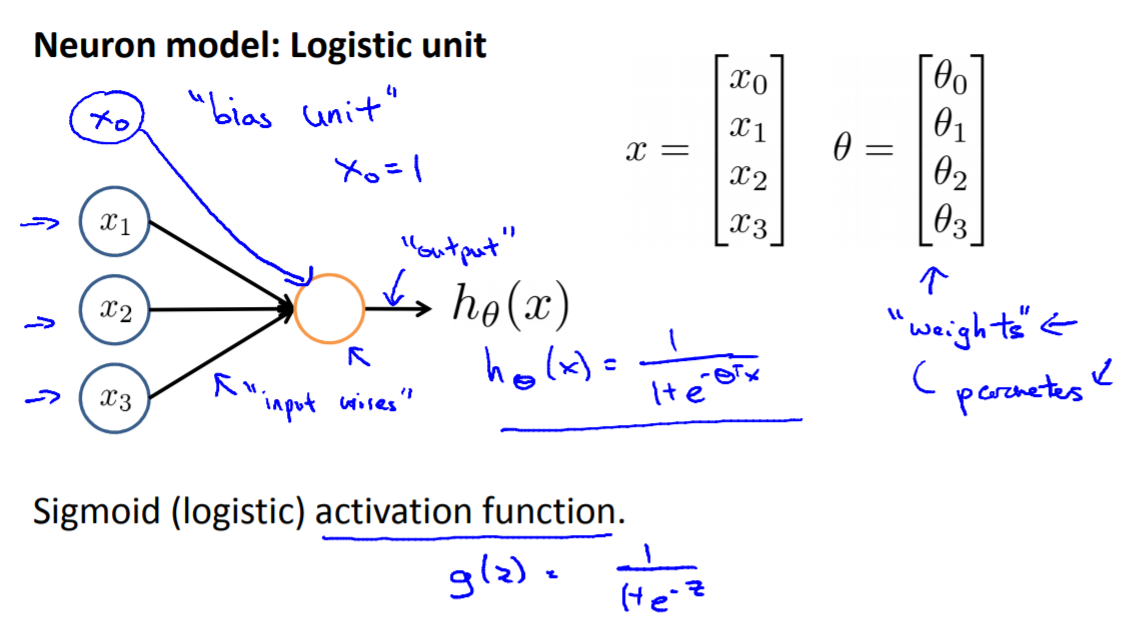## Cost Function

The Cost Function of NN is similar to that of logistic regression. The most different part is that function of NN should take output units into account, where multiple output variables can represent different classification.

In the regularization part, we should account for entire matrix of θ, including in different Units and Layers.

Logistic Regression: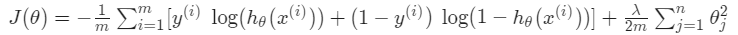Neural Network: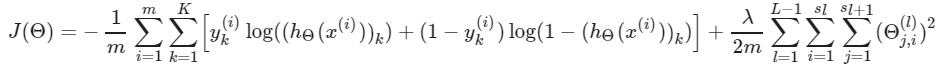Parameters

• $s_l$ = number of units (not counting bias unit) in layer l
• $s_l+1$= account for bias unit
• $x_0$= bias unit (generally set 1)
• $θ$ = weights / parameters
• $θ_{ji}$ = different manner will use ij instead of ji. It depends on you.
• $a_{ij}$ = activation of unit i in layer j
• $L$ = layers, input layer(x) → hidden layer(a) → output layer(y)
• $K$ = number of output units/classes

## Forward propagation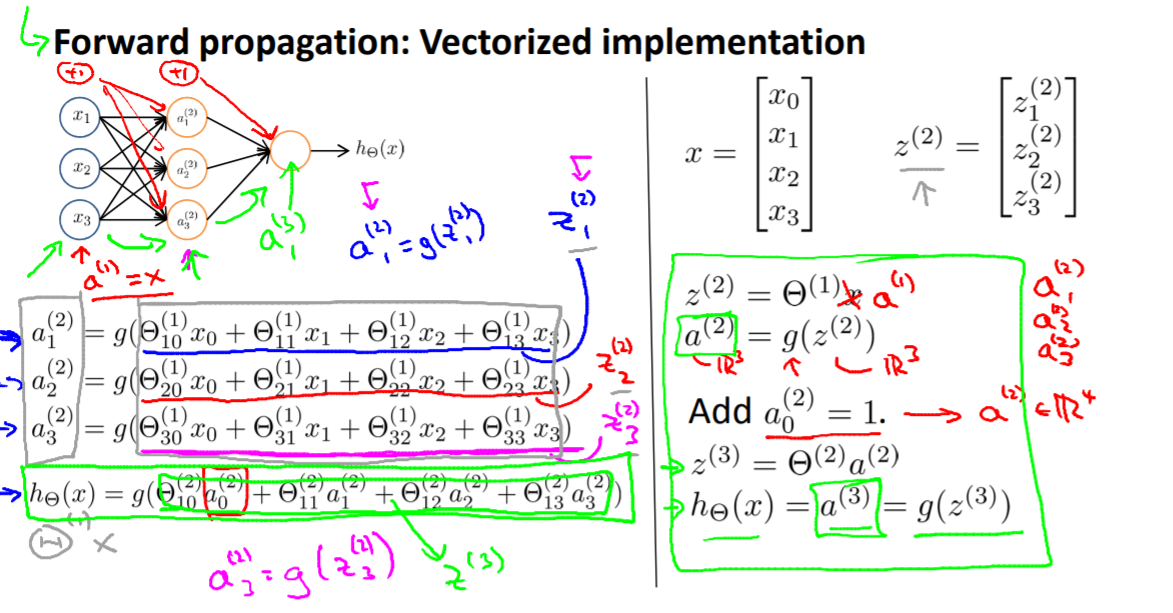Forward propagation is easily to understand and you can just write every units’ function from left to right one by one.

To summarize:

x [x1 x2 x3 x4….] → a [$a = g(θx)$ where $g(z)$ is a sigmoid/actuation function] → ai [iteration layers] → p/hθ [including $p_1 p_2 p_3...$]

note:

• $p$ = predict number which is corresponding to real number.
• When we get $h_θ$ , we also get cost function which can be minimized by gradient descent as we did before.
• When parameters are definite, using forward propagation could easily compute hypothesis function which can also predict output $y$ when $x$ are given.

# Back Propagation algorithm

As the same perception we built in linear regression and logistic regression, after we have settled cost function, our aim is to minimize cost to optimize model in order to fit the realistic data sets. That is what propagation algorithm do.

The course will introduce forward propagation(FP) and back propagation(BP) algorithm.

The propagation, in my opinion, is like computation direction. However, the difference between forward and backward does not similar to walk same path to and fro, but different in meaning, method and purpose. Concrete comparison will be stated ultimately.

## What is back propagation algorithm?

Back Propagation is really a good efficient way to make our hypothesis more accurate. The difference between forward propagation and back propagation will be discussed later.

I highly recommend an article concerning back propagation which is explicitly reveal underlying logic and relation between FP and BP. Many good examples and analogies will let you understand what how BP works. Click Here.

As we knew before, minimize our Cost function/J(θ) is ultimate purpose which means we need to compute partial derivative of $J(θ)$

Now we can use the following algorithm: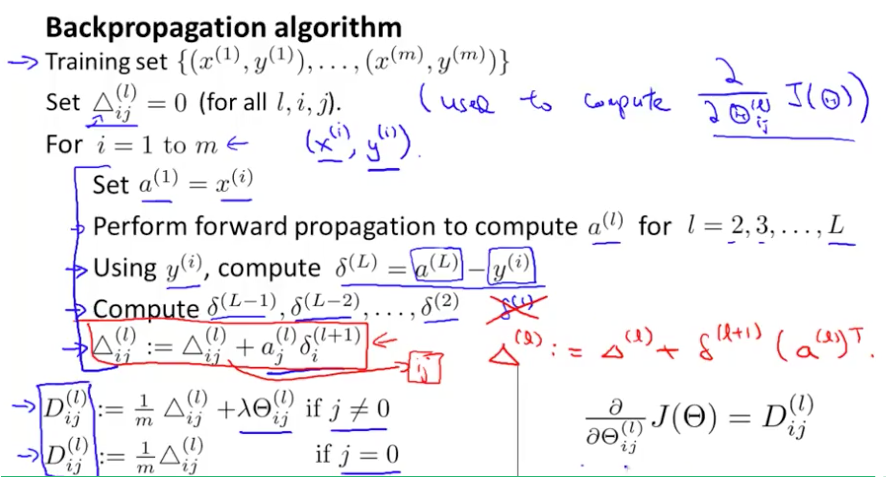The process of training data and optimizing parameters can be divided into several steps:

For training example t =1 to $m$:

1. Set: $a^{(1)} := x^{(t)}$
1. Perform forward propagation to compute $a^{(l)}$ for $l=2,3,…,L$
1. Using $y^{(t)}$, compute $\delta^{(L)} = a^{(L)} - y^{(t)}$
Where L is our total number of layers and $a^{(L)}$ is the vector of outputs of the activation units for the last layer. So our “error values” for the last layer are simply the differences of our actual results in the last layer and the correct outputs in y. To get the delta values of the layers before the last layer, we can use an equation that steps us back from right to left:
1. Compute $\delta^{(L-1)}, \delta^{(L-2)},\dots,\delta^{(2)}δ (L−1),δ (L−2),…,δ (2)$ using $\delta^{(l)} = ((\Theta^{(l)})^T \delta^{(l+1)})\ .*\ a^{(l)}\ .*\ (1 - a^{(l)})$
The delta values of layer $l$ are calculated by multiplying the delta values in the next layer with the theta matrix of layer $l$. We then element-wise multiply that with a function called g’, or g-prime, which is the derivative of the activation function g evaluated with the input values given by $z^{(l)}$.

The g-prime derivative terms can also be written out as:

1. $\bigtriangleup _{i,j}^{l} :=\bigtriangleup _{i,j}^{l}+a _{j}^{l}\cdot \delta _{i}^{l+1}$ or with vectorization,$Δ ^{l}:=Δ ^{l} +δ ^{l+1} (a ^{l} ) ^T$
Hence we update our new $\Delta$matrix.

The capital-delta matrix D is used as an “accumulator” to add up our values as we go along and eventually compute our partial derivative. Thus we get: $\frac{∂}{∂Θ _{ij}^{l}} J(Θ)= D_{ij}^{(l)}$

## Intuition and logic of BP

These algorithms seems complicated, right? Especially this function: $\delta^{(l)} = ((\Theta^{(l)})^T \delta^{(l+1)})\ .*\ a^{(l)}\ .*\ (1 - a^{(l)})$

In my opinion, this course does not clarify computing process of back propagation, thus I can not figure out how to get functions like $\delta^{(l)} = ((\Theta^{(l)})^T \delta^{(l+1)})\ .*\ a^{(l)}\ .*\ (1 - a^{(l)})$ ,which is stated above.

To better understand back propagation. Let’s go back to initial state and think about logic:

• Our purpose is to build a model( $h_θ$) to predict result.
• Consequently, cost function is implemented and we should do everything we could do to minimize cost function $J(θ)$ in order to get $θ$.
• As we did before, gradient descent is a good way to minimize it by updating weights. So, next step, we should compute partial derivative of cost function

Here we will encounter a challenge:

We need to update all parameters weights (θ) between every layers in Neural Network, where huge work needed to do.

### Chain Rule

Using a simplified example might help us understand and make a breakthrough:

When we compute partial derivative of e function, we can use c and d to represent a+b and b+1.

$e=c*d = (a+b)*(b+1)$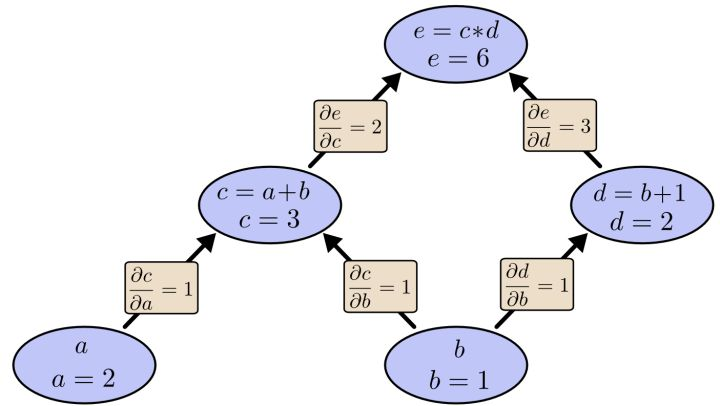Now, if want to know how a and b make impact on function e, we can compute partial derivative $\frac{\partial e}{\partial a}$ and$\frac{\partial e}{\partial b}$ through layers based on Chain Rule. The result is:

If we carefully contrast these two functions, the$\frac{\partial e}{\partial c}$ was used both in$\frac{\partial e}{\partial a}$ and$\frac{\partial e}{\partial b}$ . As units and layers grows, the communal part will be more and as for these communal parts, we can compute just once for saving computing power and increasing computing speed.

Regarding the figure shown above, we will find the second method is ‘top-down’ model while the traditional method is ‘down-top’ model. Or saying back propagation and forward propagation is similar. Concretely, we can deposit $\frac{\partial e}{\partial c} = 2$ and just use it when computing $\frac{\partial e}{\partial a}$ and$\frac{\partial e}{\partial b}$ without computing multiple times.

### Simple example

This is a Neural Network example:Parameters definition:

$J$= cost function

$a_i$ = output of unit i

$h_i$ = input of unit i

$w_(ij)$ = weigh/parameter

Firstly, if we want to compute $\frac{\partial J_{all}}{\partial w_{13}}$ as we have already know:

• $J_{all} = J_1+J_2$
• $J_{1} = \frac{1}{2}\cdot(target-a_1)^2$
• $a_1 = g(h_1)$ where $g(z)$ is actuation function(sigmoid)
• $h_1 = a_3 w_{13}+a_4w_{14}$ , ignore bias

Thus the partial derivative we want is :

Then, we can update $w_{13}$ :

It is same as computing $\frac{\partial J_{all}}{\partial w_{23}}$ , $\frac{\partial J_{all}}{\partial w_{14}}$ , $\frac{\partial J_{all}}{\partial w_{24}}$

Secondly, if we want to compute $\frac{\partial J_{all}}{\partial w_{35}}$ which is similar but a little bit different:

Thirdly, update $w_{23}^*, w_{14}^* , w_{24}^* , w_{45}^* ...$

### Inspiration

Some regularities founded while computing parameters suddenly inspire me : We have already compute $\frac{\partial J_{all}}{\partial h_3}$ and $\frac{\partial J_{all}}{\partial h_2}$ when we compute$\frac{\partial J_{all}}{\partial w_{13}}$ and $\frac{\partial J_{all}}{\partial w_{23}}$ , this part could be reused and seemed as communal part. Do you Remember that we talked in Chain Rule ? This communal part, in my opinion, might be one of most valuable points of BP.

Thus, we definite $\delta_i$ as “error”

If we vectorize our function, we can rewrite and put forward a general function equation for compute $\delta_i$:

As we process Forward propagation, we knew that:

• $h^{(l+1)} = \Theta^{(l)} a^{(l)}$
• $g ′(a(l))=\frac{\partial{a^{(l)}}}{\partial{h^{(l)}}} = a(l).∗ (1−a (l))$ when using sigmoid function as actuation function

So:

Update parameters:

If bias are taken into account, then:

# Practical Example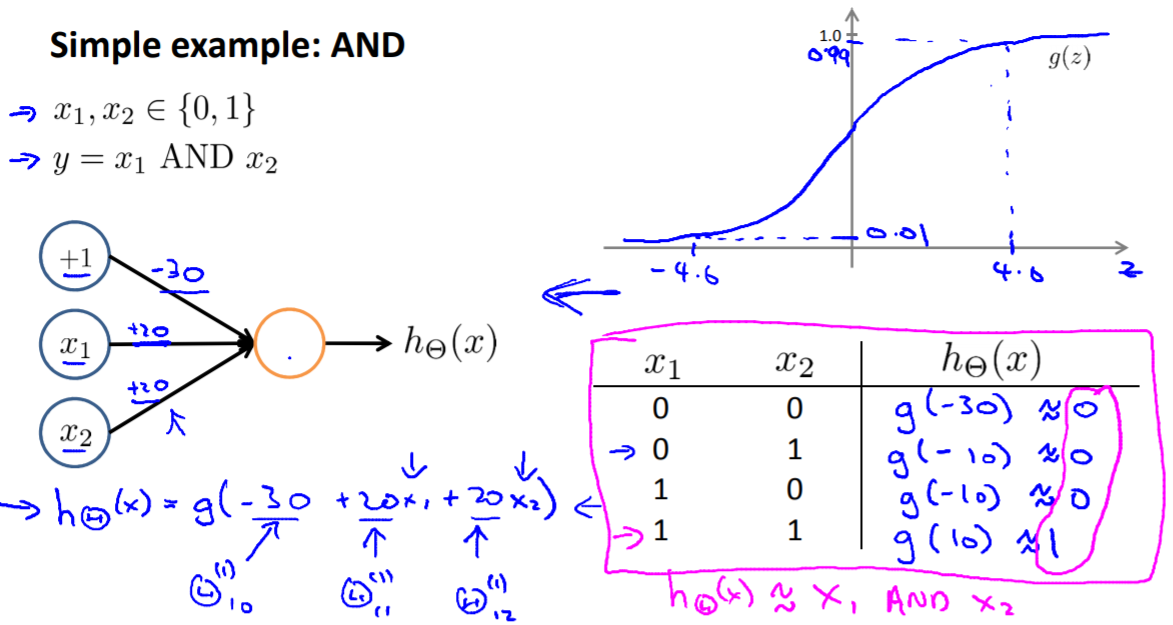Because g(z) is a sigmoid function, when we set θ as -30 or 10 (far away from -4.0 or 4.0), the y will output 0/1 as input x vary.

The simplest example is that it can indicate AND/OR/NOT/XNOR…..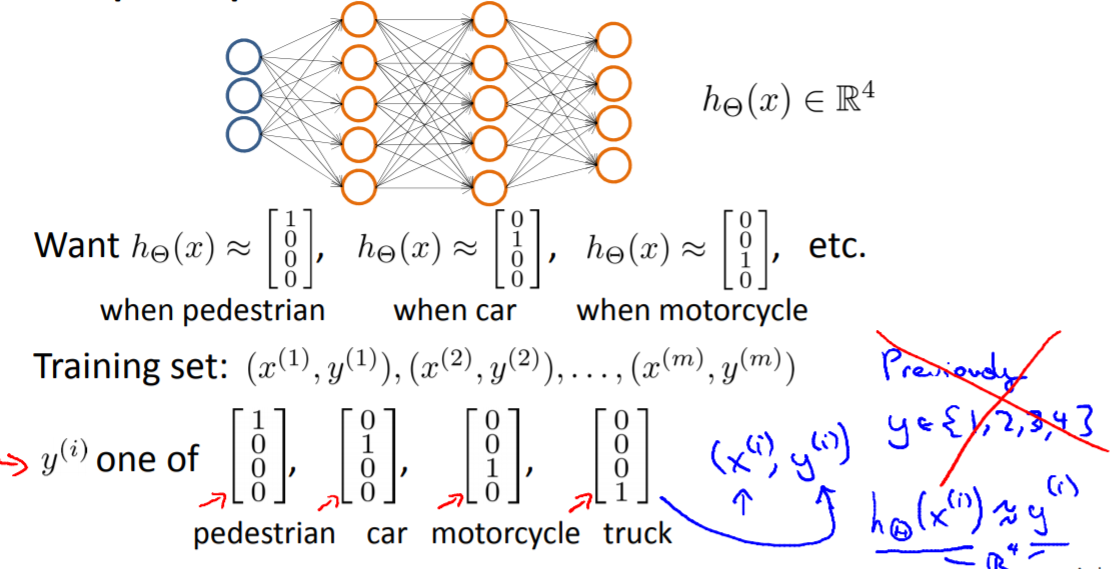When we add more output unit, the output y will indicate multiple output units, and concretely, it is binary so different y could represent different classification groups. I think examples above could extend to more output. For example, 1100/1110 may also represent some classification group.

## How to unroll parameters

thetaVector = [ Theta1(:); Theta2(:); Theta3(:); ]
deltaVector = [ D1(:); D2(:); D3(:) ]

Theta1 = reshape(thetaVector(1:110),10,11)
Theta2 = reshape(thetaVector(111:220),10,11)
Theta3 = reshape(thetaVector(221:231),1,11)


Gradient checking will assure that our backpropagation works as intended. We can approximate the derivative of our cost function with:

epsilon = 1e-4;
for i = 1:n,
thetaPlus = theta;
thetaPlus(i) += epsilon;
thetaMinus = theta;
thetaMinus(i) -= epsilon;
end;


## Random initialization

If the dimensions of Theta1 is 10x11, Theta2 is 10x11 and Theta3 is 1x11.

Theta1 = rand(10,11) * (2 * INIT_EPSILON) - INIT_EPSILON;
Theta2 = rand(10,11) * (2 * INIT_EPSILON) - INIT_EPSILON;
Theta3 = rand(1,11) * (2 * INIT_EPSILON) - INIT_EPSILON;


# Quiz:

## Week 5 /Question 5

Which of the following statements are true? Check all that apply.

1. If we are training a neural network using gradient descent, one reasonable “debugging” step to make sure it is working is to plot J(\Theta)J(Θ) as a function of the number of iterations, and make sure it is decreasing (or at least non-increasing) after each iteration.
• Correct
Since gradient descent uses the gradient to take a step toward parameters with lower cost (ie, lower J(\Theta)J(Θ)), the value of J(\Theta)J(Θ) should be equal or less at each iteration if the gradient computation is correct and the learning rate is set properly.
1. Suppose you have a three layer network with parameters \Theta^{(1)}Θ(1) (controlling the function mapping from the inputs to the hidden units) and \Theta^{(2)}Θ(2) (controlling the mapping from the hidden units to the outputs). If we set all the elements of \Theta^{(1)}Θ(1) to be 0, and all the elements of \Theta^{(2)}Θ(2) to be 1, then this suffices for symmetry breaking, since the neurons are no longer all computing the same function of the input.
• Wrong
Since the parameters are the same within layers, every unit in each layer will receive the same update during backpropagation. The result is that such an initialization does not break symmetry.
1. If we initialize all the parameters of a neural network to ones instead of zeros, this will suffice for the purpose of “symmetry breaking” because the parameters are no longer symmetrically equal to zero.
• Wrong
The trouble with initializing the parameters to all zeros is not the specific value of zero but instead that every unit in the network will get the same update after backpropagation. Initializing the parameters to all ones has the same difficulty.
1. Suppose you are training a neural network using gradient descent. Depending on your random initialization, your algorithm may converge to different local optima (i.e., if you run the algorithm twice with different random initializations, gradient descent may converge to two different solutions).
• Correct
The cost function for a neural network is non-convex, so it may have multiple minima. Which minimum you find with gradient descent depends on the initialization.
• 1316
• 1

### YOU MIGHT ALSO LIKE

0 0 vote
Article Rating
Subscribe1 评论
Inline Feedbacks